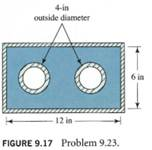### Calculate the reynolds number of the flow

Assignment Help Mechanical Engineering
##### Reference no: EM131174803

Carbon dioxide with a specific weight of 0.114 lb/ ft3 and a dynamic viscosity of 3.34 x 10-7 lb-s/ ft2 flows in the shaded portion of the duct shown in Fig. 9.17. If the volume flow rate is 200 ft3 / min, calculate the Reynolds number of the flow.#### Which project should zhe accept

The other possibility pays S20 000 now and S5000 at the end of each of the three years. In either case, his expenses will be S10 000 per year. For a ALARR of 10%, which proj

#### Determine if it is a nominal or effective rate

For each interest rate quoted, determine if it is a nominal or effective rate. If it is nominal, determine the compounding period and calculate the effective interest rate.

#### Determine the heat transfer and work transfer

Ten pounds-mass of R-22 is at an initial volume of 4.059 ft3 and pressure of 200 psia. It is compressed isobarically until its volume is two-thirds of its initial volume. The

#### What is the expected deflection on a water-mercury manometer

The flow rate of water in a 12-cm-diameter pipe is measured with a 6-cm-diameter venturi meter to be 0.09 m3 /s. What is the expected deflection on a water-mercury manometer

#### Determine the chance in capacity head and power required

The figure below shows portion of a fire protection system in which a pump draws water at 10 deg. C from a reservoir and delivers it to a point B at a flow rate of 6000 lit

#### Isotropic material-homogeneous material

Explain the following terminologies: (a) isotropic material, (b) homogeneous material, and (c) critical state. (d) Give five examples of the stress invariants you have learn

#### How does accounting differ from bookkeeping

Why is there a demand for accounting information? Name five groups that create demand for accounting information about businesses, and describe how each group uses accountin

#### Extensometer on the gauge section of a test specimen

Suppose it is impossible to use an extensometer on the gauge section of a test specimen. Instead a butt-end specimen (Figure 3.2c) is used and the strain is computed from th

### Write a Review